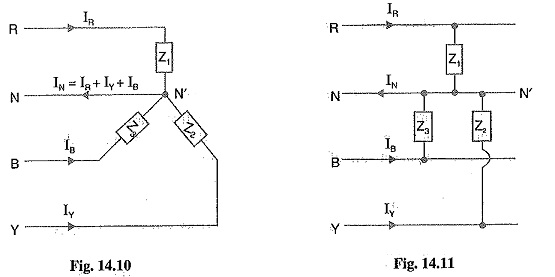The 3-phase loads that have the same impedance and power factor in each phase are called balanced loads. The problems on balanced loads can be solved by considering one phase only ; the conditions in the other two phases being similar. However, we may come across a situation when loads are unbalanced i.e. each load phase has different impedance and/or power factor. In that case, current and power in each phase will be different. In practice, we may come across the following Three Phase Unbalanced Load :

The 3-phase, 4-wire system is widely used is connected between any line and neutral wire while a 3-phase load is connected across the three lines. The 3-phase, 4-wire system invariably carries unbalanced loads. In this chapter, we shall only discuss this type of Three Phase Unbalanced Load.

We can obtain this type of load in two ways. First, we may connect a 3-phase, 4-wire unbalanced load to a 3-phase, 4-wire supply as shown in Fig. 14.10. Note that star point N of the supply is connected to the load star point N’. Secondly, we may connect single phase loads between any line and the neutral wire as shown in Fig.14.11. This will also result in a 3-phase, 4-wire unbalanced load because it is rarely possible that single phase loads on all the three phases have the same magnitude and power factor. Since the load is unbalanced, the line currents will be different in magnitude and displaced from one another by unequal angles, The current in the neutral wire will be the phasor sum of the three line currents i.e.

Current in neutral wire,The following points may be noted carefully

1.Since the neutral wire has negligible resistance, supply neutral N and load neutral N’ will be at the same potential. It means that voltage across each impedance is equal to the phase voltage of the supply. However, current in each phase (or line) will be different due to unequal impedances.

2.The amount of current flowing in the neutral wire will depend upon the magnitudes of line currents and their phasor relations. In most circuits encountered in practice, the neutral current is equal to or smaller than one of the line currents. The exceptions arc those circuits having severe unbalance.

Scroll to Top